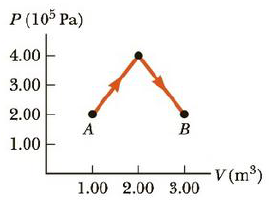Chapter 12, Problem 76AP

Chapter
Section
Textbook Problem

A diatomic ideal gas expands from a volume of VA = 1.00 m3 to VB = 3.00 m3 along the path shown in Figure P12.76. If the initial pressure is PA = 2.00 × 105 Pa and there are 87.5 mol of gas, calculate (a) the work done on the gas during this process, (b) the change in temperature of the gas, and (c) line change in internal energy of the gas. (d) How much thermal energy is transferred to the system?Figure P12.76

(a)

To determine
The work done on the gas during the process.

Explanation

Given Info:

The width of rectangle is 2m3 .

The height of rectangle is 2×105Pa

The base of triangle is 2m3 .

The height of the triangle is 2×105Pa

The area under the graph in a PV diagram is equal in magnitude to the work done on the substance. If the finial volume is greater than the initial volume, then the work done on the substance is negative.

Formula to calculate the area is,

A=(W1×H1)+12(B1×H2)

• W1 is the width of rectangle
• H1 is the height of rectangle
• B1 is the base of triangle
• H2 is the height of triangle

Substitute 2m3<

(b)

To determine
The change in temperature of the gas.

(c)

To determine
The change in internal energy of the gas.

(d)

To determine
How much energy is transferred to the system.

Still sussing out bartleby?

Check out a sample textbook solution.

See a sample solution

The Solution to Your Study Problems

Bartleby provides explanations to thousands of textbook problems written by our experts, many with advanced degrees!

Get Started